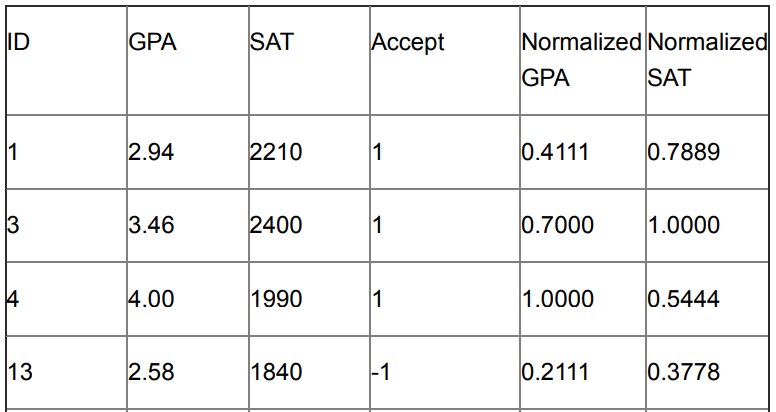Search

# What Is Support Vector Machine(SVM) In Machine Learning?

• Support Vector Machines (SVMs) are supervised learning techniques that analyze data and recognize patterns.

• SVMs can be applied to both classification and numeric prediction problems.

• The basic idea of SVM for classification is to construct a hyperplane (or a set of hyperplanes) that best separates the data points of different classes.

• SVMs can be non-linear, but it is very difficult to get any intuition for non-linear SVMs.

Here the Given format of given dataset### SVM Classification

SVMs are a useful technique for data classification(predicting categorical variable). Even though other methods are thought to be easier to use than an SVM, sometimes these produce unsatisfactory results. The goal of an SVM is to produce a model that can predict the target value of data instances in the testing set, given only the attributes.

Classification in an SVM is an example of supervised learning. Known labels help indicate whether the system is performing correctly or not. This information points to a desired response, validating the accuracy of the system — or which can be used to help the system learn to act correctly.

### SVM Regression

SVMs can also be applied to regression problems(predicting numerical variable). Similarly to classification problems, a nonlinear model is usually required to adequately model data. In the regression method, there are considerations based on prior knowledge of the problem and the distribution of the noise to choose some adjustable parameters.

### Mathematical Formula

We now use an example to describe how to find the center hyperplane algebraically for linear svms.

For the above dataset we need to find a line (the mid-line in the shaded area) equation.Given the table of dataset, we first set C=1000, which is an adjustable parameter. we notice it has 7 rows, 2 independent variables....

...We then minimize the above function under the 7 conditionsNote: the first 3 conditions are corresponding the first 3 rows of the dataset and the last 4 conditions are corresponding to the last 4 rows of the dataset (where y appears as the first coefficient in all the conditions), also note how 𝑥1, 𝑥2 are used inis not the minimizer.

It turns out that:### Python Implementation Using Sklearn

```#Import Libraries
import numpy as np
import matplotlib.pyplot as plt
from sklearn import svm
import pandas as pd

```
```#Read Dataset
X=my_data[['Normalized GPA', 'Normalized SAT']]
y=my_data['Accept']```
```#Split Dataset
from sklearn.model_selection import train_test_split
X_train, X_test, y_train, y_test = train_test_split(X, y, test_size=0.2, random_state=0)```
```#Finding the score
for my_C in [1,5,10,20,100,1000]:
clf = svm.SVC(kernel='rbf', C=my_C)
clf.fit(X_train, y_train)
print("C=%f score=%f" %(my_C,clf.score(X_test, y_test)))```

### Output:And get instant help in any other machine learning related help.

### You Might Get Help In

• Machine Learning Assignment Help

• Deep Learning Assignment Help

• Data Mining Assignment Help

• Big Data Assignment Help

• And More Others